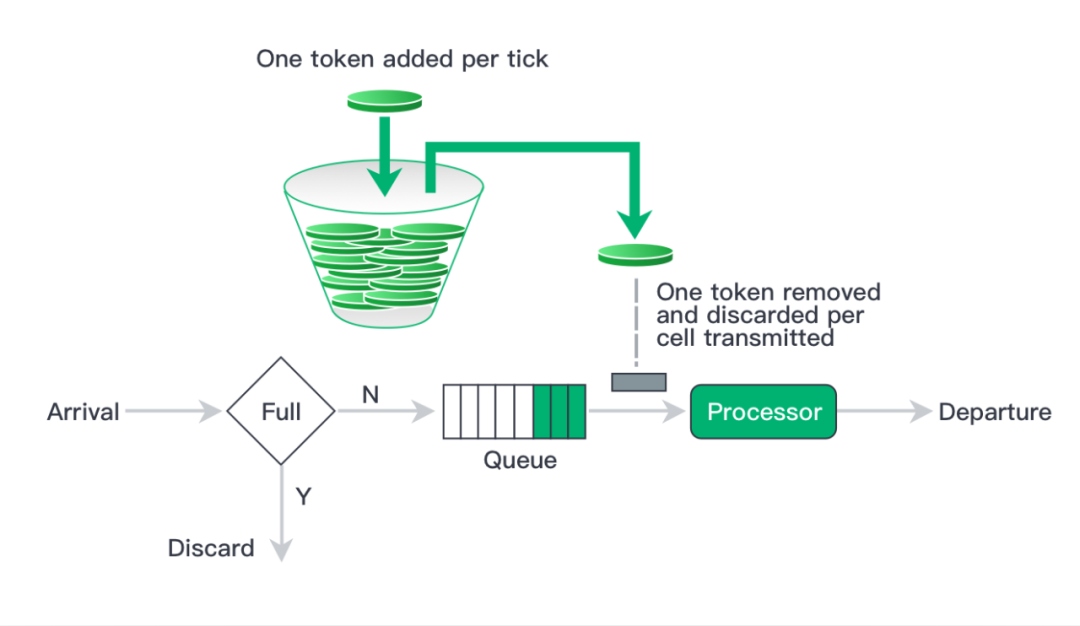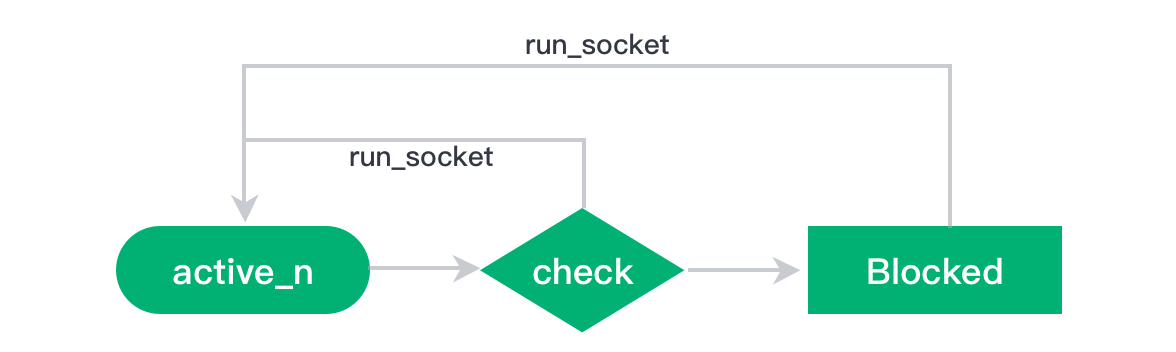Upcoming Webinar: MQTT over QUIC: A New Standard for Connected Vehicles
Register Now →

# The configuration guide of EMQX Rate LimitEMQX Team Jul 30, 2020Before reading this guide, we assume that you have known simple MQTT and MQTT broker.

EMQX Broker starts supporting the function rate limit from version V3, including the limit on the PUBLISH packet receiving rate and the TCP data package receiving rate. This article will introduce the use and configuration of this function in detail.

## Configuration items

### MQTT PUBLISH packet receiving rate

This configuration locate on `emqx.conf`:

`zone.external.publish_limit = 10,1m`

The format of configuration is: `<Number>,<Duration>`, which means the maximum number `<Number>` of PUBLISH packet that can be allowed to receive during the time `<Duration>`.

### TCP data package receiving rate

This configuration locate on `emqx.conf`:

`listener.tcp.external.rate_limit = 1024,4096`

The format of configuration is: `<Rate>,<Burst>`, which means the allowed average data package receiving rate is `<Rate>`. However, the allowed maximum number is depending on the value of `<Burst>`. See the next section for details: rate limit algorithm token bucket-algorithm

### active_ n

This configuration locate on `emqx.conf`:

`listener.tcp.external.active_n = 100`

`active_n` actually means that the number of datagrams allowed to be read in the underlying asynchronous I/O. Every time, when the asynchronous read operation reaches this limit, it will be temporarily switched to the Sync mode. Every time, when it switches to the Sync mode, it will check the rate limit one time. Therefore, if the value is larger, the throughput performance of this system is better; if the value is smaller, rate checking is more accurate and the inflow is more stable and the security of the system is higher.

## Rate limit algorithm - token bucket

### Introduction

The `publish_limit` and `rate_limit` we mentioned are implemented by the token bucket algorithm. The algorithm logic is as follows:1. There is a bucket of a token that can hold up the number of `burst` at most.
2. We will add tokens into this bucket at a rate of N per second. If the bucket is full, no more tokens are added to this bucket. We call this rate as `rate`.
3. When there are requests, we will take the corresponding number of tokens from the bucket. If the bucket is empty, block it until enough tokens are put in.

### Function

Through token bucket algorithm, we can:

• In the case of running for a long time, the average value of the limited request rate is equal to the rate of adding tokens, the `rate`.
• Allow a certain level of peak flow. If the request rate is `M` and is greater than `rate`, the rate of token decreasing is `M - rate`. Therefore, the time for us to take all the tokens from a full bucket is `burst / (M - rate)`, and the number of the requests be accepted is `burst / (M - rate) * M` during this period.

All in all, it can be simply understood as `rate` is the average request rate and `burst` is is the instantaneous maximum request rate.

## The implementation of EMQX rate limit

Based on the token bucket algorithm, EMQX's implementation logic for rate limit is as follows:Its meaning is:

1. The socket performs a rate checking every time it receives n TCP data packets, and will record the total length of the received n TCP data packets as s.
2. If the number of left tokens in the bucket is greater and equal to s, will update the number of tokens on the corresponding bucket and active_n will be performed through continue activating socket.
3. If the number of left tokens in the bucket r is less than s, will active socket after waiting for `(s - r) / rate` seconds.

## The example of rate limit configuration

### The configuration of rate limit

``````listener.tcp.external.active_n = 100
listener.tcp.external.rate_limit = 1024,1024000
``````

The above configuration means:

• Check the rate every 100 TCP packets are received.
• The average rate limit is 1024 byte/s.
• Bucket size is 1000KB. If the total length of this 100 TCP packets is greater than 1000KB, the rate limit will be triggered.

Therefore, users need to set `<Burst>` according to the size of the real packet. EMQX highly recommends that configure it as `(max_packet_size * active_n) / 2` to prevent from block.

``````listener.tcp.external.active_n = 100
zone.external.publish_limit = 10,1m
``````

The above configuration means:

• Check the rate every 100 TCP packets are received.
• Only 10 PUBLISH packet will be allowed to receive within one minute.

You can transfer `<Number>,<Duration>` into the form of `<Rate>,<Burst>`, namely `<Number> / <Duration>, <Numebr>`.

### Other configurations

In addition to the above Rate Limit, it is also supported that perform the following limits for the TCP, WebSocket and other connections:

`listener.tcp.external.max_connections = 1024000`

The maximum connection number that exists at the same time allowed, namely the maximum number of simultaneous online clients.

`listener.tcp.external.max_conn_rate = 1000`

The maximum number of concurrent connections allowed per second.